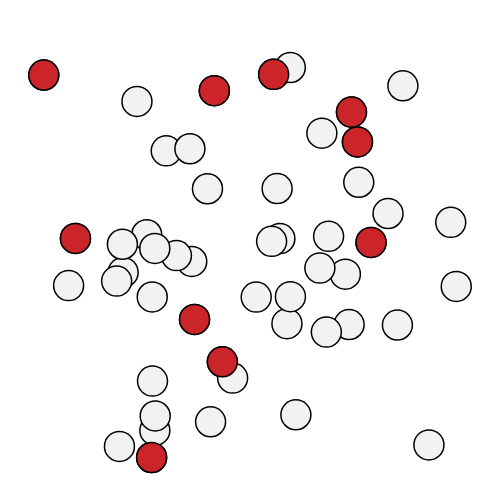# sf.apps.plot.points¶

points(R, sample=None, plot_size=(500, 500), point_size=30)[source]

Creates a plot of two-dimensional points given their input coordinates. Sampled points can be optionally highlighted among all points.

Example usage:

>>> R = np.random.normal(0, 1, (50, 2))
>>> sample =  * 10 +  * 40  # select first ten points
>>> plot.points(R, sample).show()Parameters
• R (np.array) – Coordinate matrix. Rows of this array are the coordinates of the points.

• sample (list[int]) – optional subset of sampled points to be highlighted

• plot_size (int) – size of the plot in pixels, given as a pair of integers (x_size, y_size)

• point_size (int) – size of the points, proportional to its radius

Returns

figure of points with optionally highlighted sample

Return type

Figure